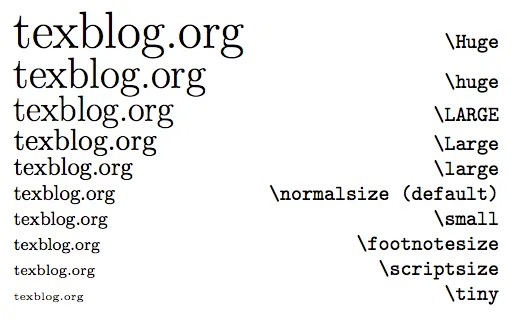### 写在前面

咱觉得Markdown的语法比较乱套,只能看用的哪个编辑器具体讨论,以下内容仅供参考,因为放到具体环境中能否work谁也不知道,但是大多数情况下应该还是OK的.

之前这个问题困扰了咱一段时间,因为画出来的公式不好看感到很不满意,今天查了下发现不需要什么邪招,方法还是很多的.大多数的问题都是被Typora坑了,如果有编辑器不支持的方法就尝试换个表达方式看看,兴许有奇效.

### 字体大小

1. 之前一直尝试的方法, 在某些编辑器中行不通之后以为不支持, 结果后来发现只是编辑器的锅, 大多数Markdown解析器还是可以用的:

1<font size=32>$E=mc^2$</font>


效果大概像是这个样子:

E=mc^2

2. 另外还有一个公式本身就支持的方式, 咱比较推荐用这个.

1$$2 \Large E=mc^2 3$$


效果大概像是这个样子:

\Large E=mc^2

其中这个Large可以替换成很多别的规格,尝试用表格码出来发现难度挺高,贴个图凑活下吧.### 粗体斜体

一个有意思的事情是,咱在手头的编辑器中尝试使用星号改变字体统统失败了,猜测渲染公式的字体和Markdown用的不是一套, 可以用下面这个方法(虽然除了要用黑体以外,公式的字体一般不用改,我觉得挺好看的).

Solo用的KaTeX貌似没有mit(数学斜体)这个字体, 和默认字体没啥区别就不展示了.

\rm 罗马体 \rm{E=mc^2}
\it 意大利体(默认) \it{E=mc^2}
\bf 粗体 \bf{E=mc^2}
\boldsymbol 黑体 \boldsymbol{E=mc^2}
\Bbb 黑板粗体 (-\infty,+\infty)=\Bbb{R}

### 换行 居中

我知道这个问题巨蠢, 但说到底还是被坑到怀疑人生的产物罢了.

1. 单行公式使用"$$...$$", 行内公式用"$...$"

1$E=mc^2$
2$$3 E=mc^2 4$$


效果大概像是这个样子:

E=mc^2

E=mc^2

2. 公式内换行使用"\\",对齐使用"align"

公式内换行直接用就行, 这里额外介绍下align的用法, 这玩意儿是个环境, 需要用如下所示的方法启用,其中"&"表示对齐,"\\"同样是表示换行.

12 \begin{align} 3 x^2-8 & = 2x \\ 4 x^2-2x+1 & = 9 \\ 5 (x-1)^2 & = (\pm3)^2 6 \end{align} 7

\begin{aligned} x^2-8 & = 2x \\ x^2-2x+1 & = 9 \\ (x-1)^2 & = (\pm3)^2 \end{aligned}

对于有的编辑器提示no such environment时可以尝试使用"aligned",比如手头这个.

• 这回是来造访下强哥的博客, 感觉很有趣! 强哥的博客也和强哥一样强, 心满E足了.
• 这篇文章中的缩进用的是&emsp;的方法,有没有更加简单点的,Solo上制表或者8个空格都会变成代码块,是不是我用的方法有什么问题? 后来意识到这个缩进并不是那么重要, 使用两个换行生成的段落间隔可以很清晰地分开两段文字, 推荐使用这个方法.
• 此外咱习惯用中文输入法外加英文标点去码字,可能是代码敲多了习惯性加空格的毛病犯了.但是在Solo中,英文标点被渲染成了中文标点,这...有点别扭.特别是英文括号后面的句号却保留为半角标点符号,极度难受. 可以在配置中关闭这一选项, 还挺人性化的.
• 此外发现Solo的编辑器中使用单行公式(用两个\$符号扩起来的那个)时,在空白情况下backspace会莫名复制出一对的bug,比较魔幻,希望能有改进吧.
• 完事儿以后表格编辑中按回车会把整个表格给我把整个表格的内容复制一遍,可以自己体会一下,比较影响使用体验. 这个bug貌似是修复了? 可喜可贺.